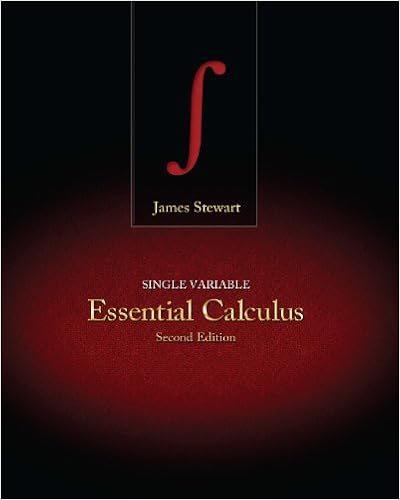# B assume that the length of the conjugated portion of

• Test Prep
• 10
• 100% (20) 20 out of 20 people found this document helpful

This preview shows page 5 - 8 out of 10 pages.

##### We have textbook solutions for you!
The document you are viewing contains questions related to this textbook.The document you are viewing contains questions related to this textbook.
Chapter 2 / Exercise 54
Single Variable Essential Calculus
StewartExpert Verified
10B) Assume that the length of the conjugated portion of retinal is 15 Å, what wavelength of light would you expect to cause an electron to transition to the lowest unoccupied energy state? (10 points)
##### We have textbook solutions for you!
The document you are viewing contains questions related to this textbook.The document you are viewing contains questions related to this textbook.
Chapter 2 / Exercise 54
Single Variable Essential Calculus
StewartExpert Verified
A 6 10C) Assuming the change in energy above for retinal, what would the uncertainty be for the time scale of the electronic transition? (5 points)
10D) Assume you wanted to engineer the wavelength of the light that opens (activates/gates) ChR, to be twice that above (9B), how would that affect the length of the ChR retinal cofactor? (Hint: a simple mathematical expression is sufficient, no need to calculate). (5 points)
11A) Nitrogenases are enzymes that catalyzes nitrogen gas (N2) fixation converting it to either ammonium (NH4+) or nitrogen dioxide (NO2). These nitrogen based components are essential in biology ultimately ending up in nucleotides (DNA, RNA) and amino acids (proteins). In an effort to begin to dissect a specific nitrogenase mechanism, you measure the vibrational frequency (wavenumber) of 14N2to be 2327 cm-1. What is the spring constant for nitrogen gas (14N2)? (10 points).
��
𝑚
A 7
•••